# Angles

Angle. Degree and radian measures of an angle.
Right (direct), acute and obtuse angle. Mutually
perpendicular straight lines. Signs of angles.
opposite (vertical) angles. Bisector of an angle.

Angle is a geometric figure  ( Fig.1 ),  formed by two rays  OA  and  OB ( sides of an angle ), going out of the same point  O  (a vertex of an angle ).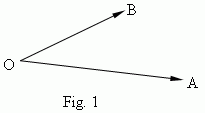An angle is signed by the symbol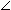and three letters, marking ends of rays and a vertex of an angle:AOB (moreover, a vertex letter is placed in the middle). A measure of an angle is a value of a turn around a vertex O, that transfers a ray OA  to the position OB. Two units of angles measures are widely used: a radian and a degree . About a radian measure see below in the point "A length of arc" and also in the section "Trigonometry" .
A degree measure. Here a unit of measurement is a degree ( its designation is ° or deg ) a turn of a ray by the 1/360 part of the one complete revolution. So, the complete revolution of a ray is equal to 360 deg. One degree is divided by 60 minutes ( a designation is ‘ or min ); one minute – correspondingly by  60 seconds ( a designation is “ or sec ).An angle of  90 deg  ( Fig.2 ) is called a right or direct angle; an angle lesser than  90 deg  ( Fig.3 ), is called an acute angle; an angle greater than  90 deg  ( Fig.4 ), is called an obtuse angle.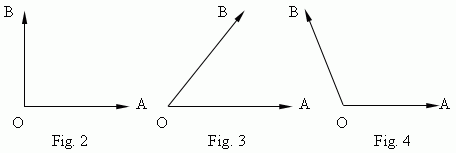Straight lines, forming a right angle, are called mutually  perpendicular lines. If  the straight lines AB and MK are perpendicular, this is signed as: AB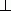MK.

Signs of angles. An angle is considered as positive , if a rotation is executed opposite a clockwise , and negative – otherwise. For example, if the ray OA displaces to the ray OB as shown on  Fig.2, thenAOB = + 90 deg; but on Fig.5AOB = – 90 deg.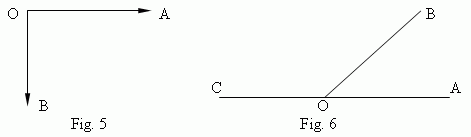Supplementary (adjacent) angles ( Fig.6 ) – angles AOB and COB, having the common vertex O and the common side OB;

other two sides OA and OC form a continuation one to another. So, a sum of supplementary (adjacent) angles is equal to 180 deg.
Vertically opposite (vertical) angles ( Fig.7) – such two angles with a common vertex, that sides of one angle are continuations of the other:AOB andCOD ( and alsoAOC andDOB )  are vertical angles.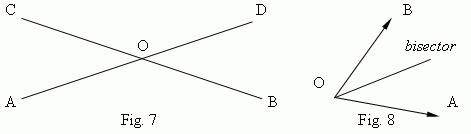A bisector of an angle is a ray, dividing the angle in two ( Fig.8 ). Bisectors of vertical angles (OM and ON, Fig.9) are continuations one of the other. Bisectors of supplementary angles (OM and ON, Fig.10) are mutually perpendicular lines.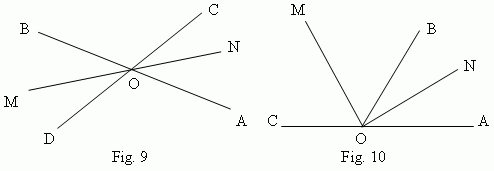The property of an angle bisector: any point of an angle bisector is placed by the same distance from the angle sides .